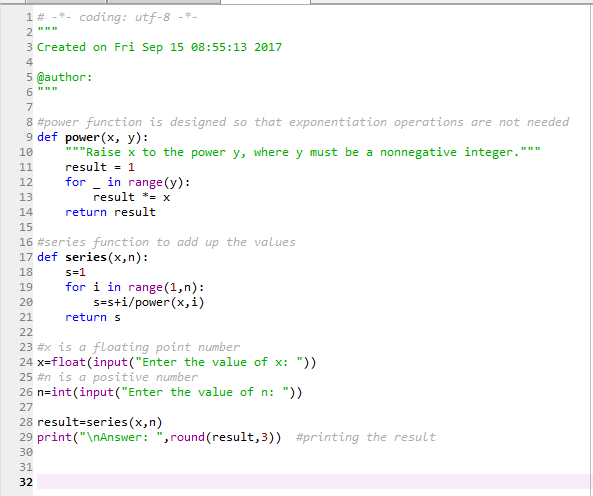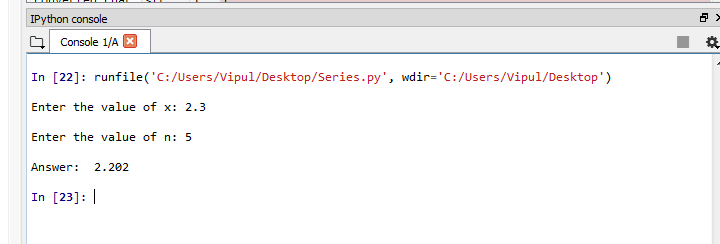# Question & Answer: *Code language is in Python for reference……

*Code language is in Python for reference.

For each of the problems below state the purpose in your own words, specify input that is needed, expected output, and the step by step process that will obtain the output from the input (the algorithm). In addition to these 4 items also specify test data that can be used for each problem. Remember to describe your steps in enough detail so someone other than you could follow your algorithm and solve the problem. Also do not write any actual code.

Don't use plagiarized sources. Get Your Custom Essay on
Question & Answer: *Code language is in Python for reference……
GET AN ESSAY WRITTEN FOR YOU FROM AS LOW AS \$13/PAGE

Problem 1: Create a process that will calculate the series 1 + 1/x + 2/x^2 + 3/ x^3 + 4/x^4 ………. + n/x^n where x is a floating point number and n is a positive number. Your process should be designed so exponentiation operations are not needed.

Problem 2: Write a process that will complete will find the sum of the following series. 1/n + 2/(n-1) + 3/(n-2) + ………. + n/1 Program 3: Write a process to determine all the whole numbers that would evenly divide an entered whole number. Think about how to minimize the number divisions needed.

Program 3: Write a process to determine all the whole numbers that would evenly divide an entered whole number. Think about how to minimize the number divisions needed.

This is long assignment to do at once. Please post the other questions seperately. I am providing you the solution for Problem1. Let me know if you have any doubt.

Purpose: Finding the sum of series of a given value and number of terms.
Input : 2 input requires. One is float value other is integer value
Output: Output will be the sum of terms uptu n.
Steps: steps are there in the program

#power function is designed so that exponentiation operations are not needed
def power(x, y):
“””Raise x to the power y, where y must be a nonnegative integer.”””
result = 1
for _ in range(y):
result *= x
return result

#series function to add up the values
def series(x,n):
s=1
for i in range(1,n):
s=s+i/power(x,i)
return s

#x is a floating point number
x=float(input(“Enter the value of x: “))
#n is a positive number
n=int(input(“Enter the value of n: “))

result=series(x,n)